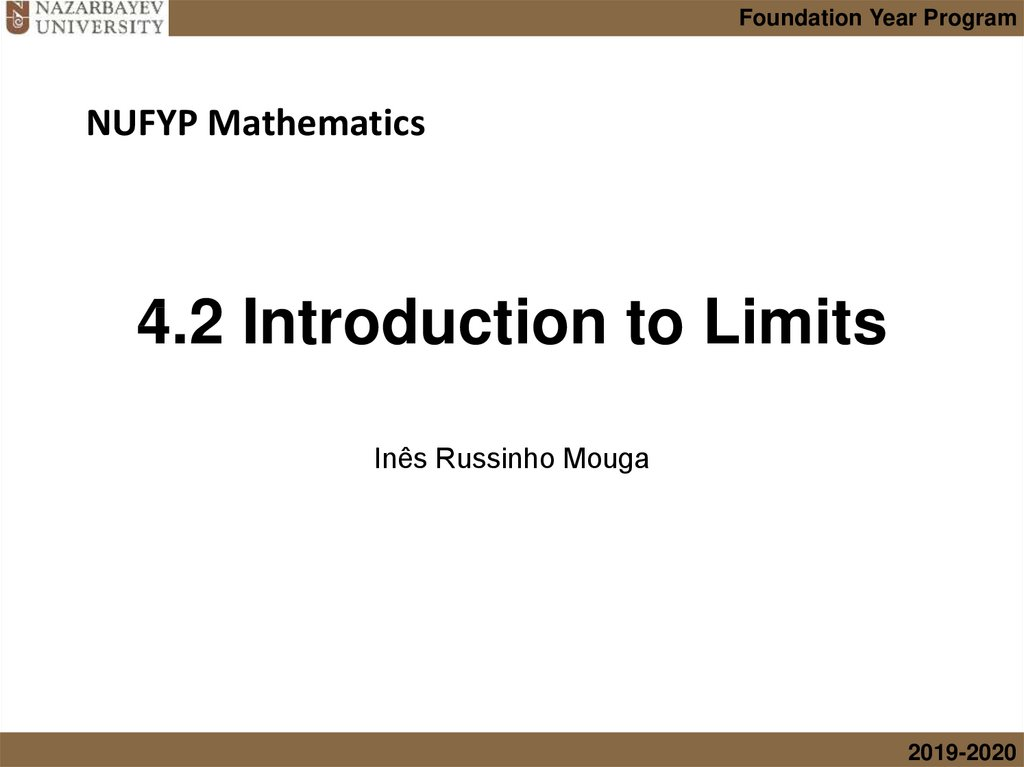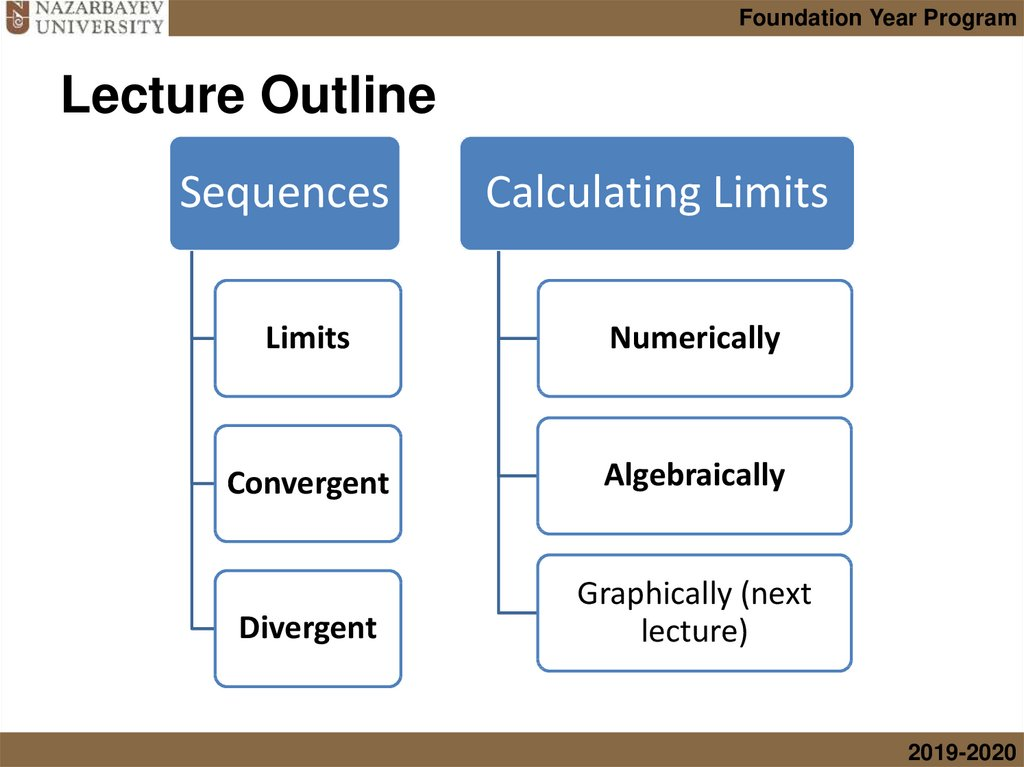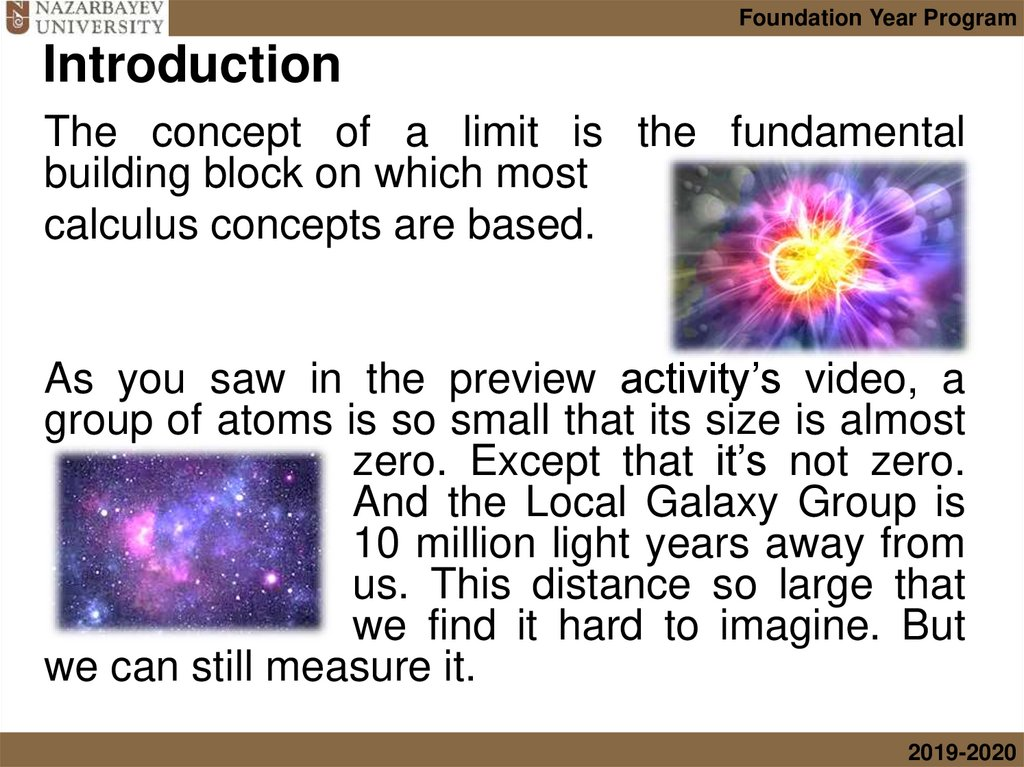# 4.2 Introduction to Limits

## 1. 4.2 Introduction to Limits

Foundation Year Program
NUFYP Mathematics
4.2 Introduction to Limits
Inês Russinho Mouga
2019-2020

## 2. Lecture Outline

Foundation Year Program
Lecture Outline
Sequences
Calculating Limits
Limits
Numerically
Convergent
Algebraically
Divergent
Graphically (next
lecture)
2019-2020

## 3. Introduction

Foundation Year Program
Introduction
The concept of a limit is the fundamental
building block on which most
calculus concepts are based.
As you saw in the preview activity’s video, a
group of atoms is so small that its size is almost
zero. Except that it’s not zero.
And the Local Galaxy Group is
10 million light years away from
us. This distance so large that
we find it hard to imagine. But
we can still measure it.
2019-2020

## 4. Introduction

Foundation Year Program
Introduction
There is no end to the natural numbers. Say
the largest number you can imagine, the
colleague sitting next to you will say the
same number plus one, and that will be a
larger number. And we can go on and on
forever…
2019-2020

## 5. Infinitely large…

Foundation Year Program
Infinitely large…
Let’s consider the sequence of even numbers